# Electric Current and Direct Current Circuits Electric Current

• Slides: 34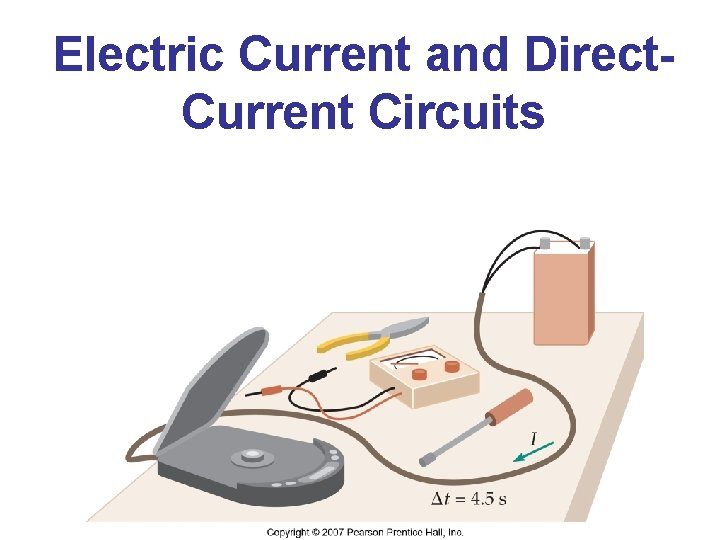Electric Current and Direct. Current Circuits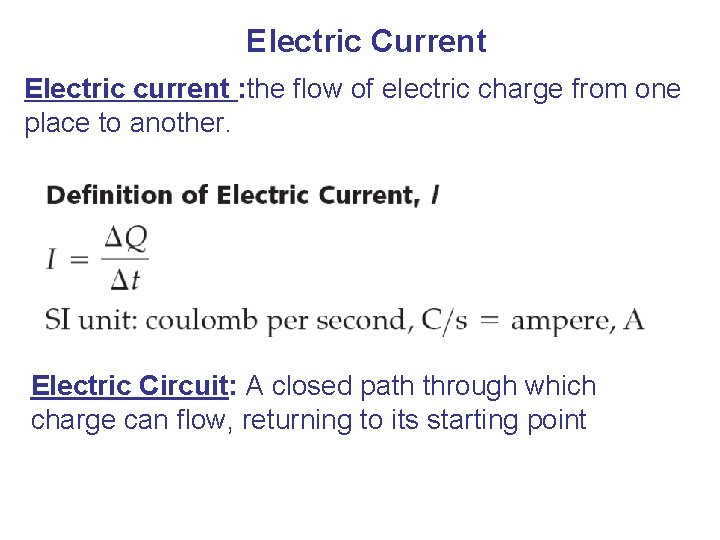Electric Current Electric current : the flow of electric charge from one place to another. Electric Circuit: A closed path through which charge can flow, returning to its starting point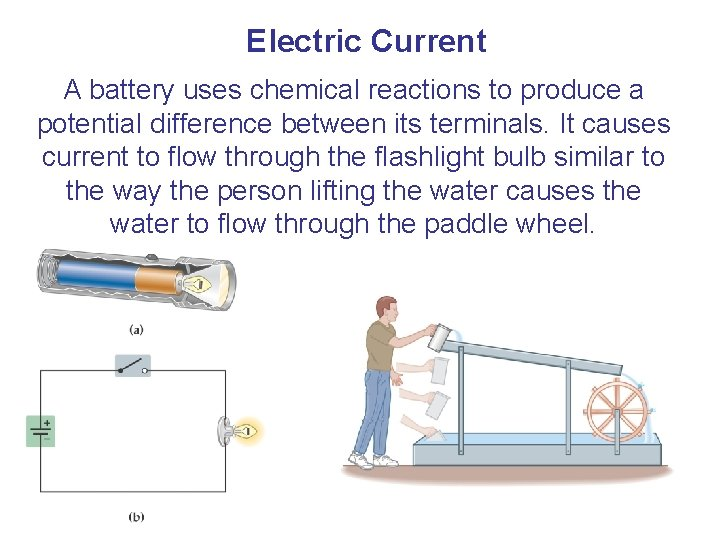Electric Current A battery uses chemical reactions to produce a potential difference between its terminals. It causes current to flow through the flashlight bulb similar to the way the person lifting the water causes the water to flow through the paddle wheel.Electric Current A battery that is disconnected from any circuit has an electric potential difference between its terminals that is called the electromotive force or emf: Remember – despite its name, the emf is an electric potential, not a force. The amount of work it takes to move a charge ΔQ from one terminal to the other is: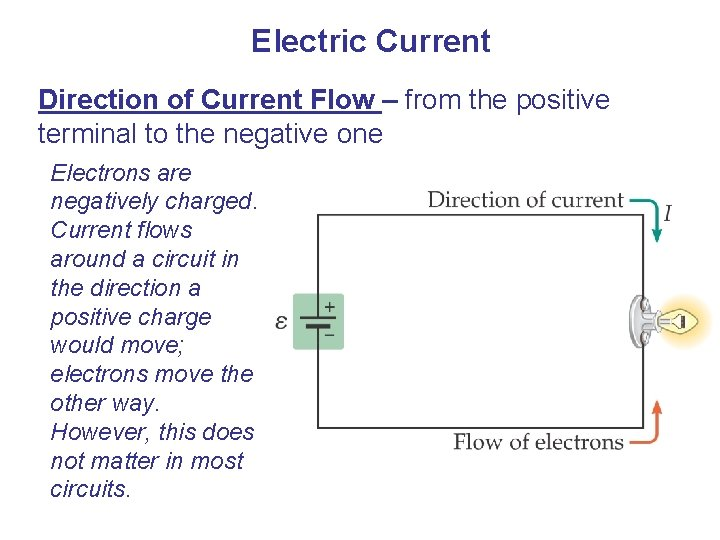Electric Current Direction of Current Flow – from the positive terminal to the negative one Electrons are negatively charged. Current flows around a circuit in the direction a positive charge would move; electrons move the other way. However, this does not matter in most circuits.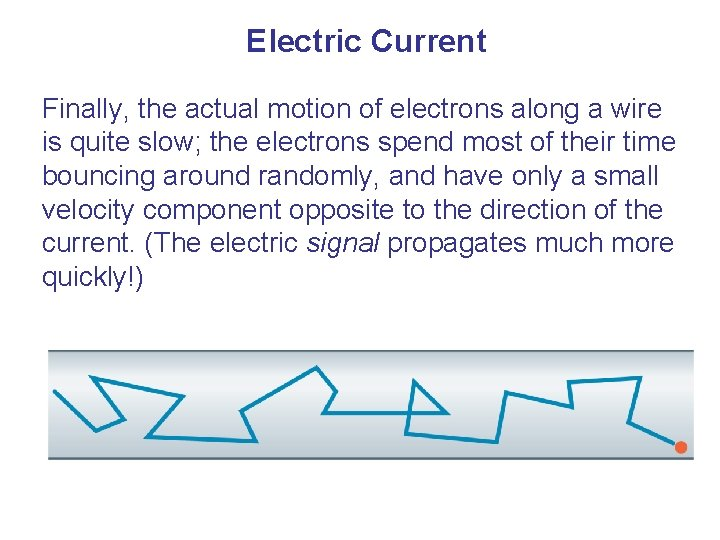Electric Current Finally, the actual motion of electrons along a wire is quite slow; the electrons spend most of their time bouncing around randomly, and have only a small velocity component opposite to the direction of the current. (The electric signal propagates much more quickly!)Resistance and Ohm’s Law Under normal circumstances, wires present some resistance to the motion of electrons. Ohm’s law relates the voltage to the current: Be careful – Ohm’s law is not a universal law and is only useful for certain materials (which include most metallic conductors).Resistance and Ohm’s Law Solving for the resistance, we find The units of resistance, volts per ampere, are called ohms:Resistance and Ohm’s Law Two wires of the same length and diameter will have different resistances if they are made of different materials. This property of a material is called the resistivity.Resistance and Ohm’s Law The difference between insulators, semiconductors, and conductors can be clearly seen in their resistivities: See Table 17. 1 pg. 538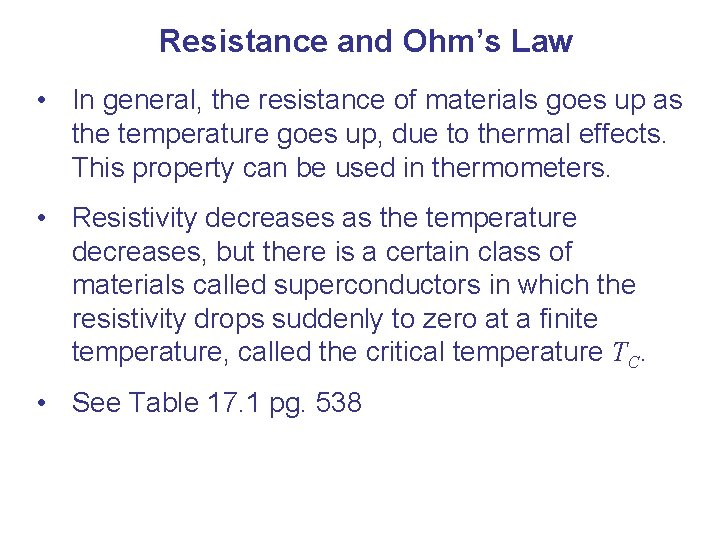Resistance and Ohm’s Law • In general, the resistance of materials goes up as the temperature goes up, due to thermal effects. This property can be used in thermometers. • Resistivity decreases as the temperature decreases, but there is a certain class of materials called superconductors in which the resistivity drops suddenly to zero at a finite temperature, called the critical temperature TC. • See Table 17. 1 pg. 538Energy and Power in Electric Circuits When a charge moves across a potential difference, its potential energy changes: Therefore, the power it takes to do this is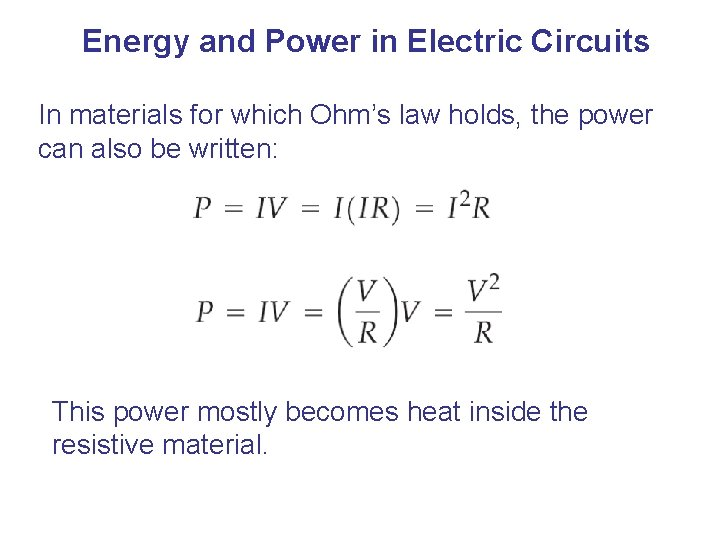Energy and Power in Electric Circuits In materials for which Ohm’s law holds, the power can also be written: This power mostly becomes heat inside the resistive material.Energy and Power in Electric Circuits When the electric company sends you a bill, your usage is quoted in kilowatt-hours (k. Wh). They are charging you for energy use, and k. Wh are a measure of energy.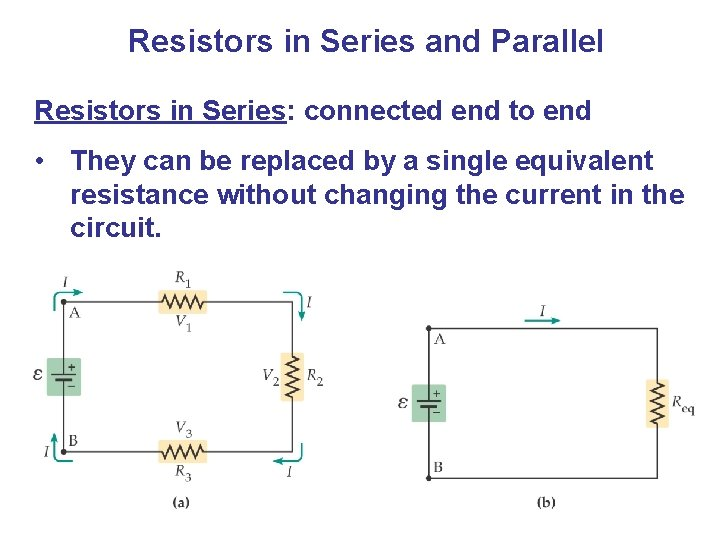Resistors in Series and Parallel Resistors in Series: connected end to end • They can be replaced by a single equivalent resistance without changing the current in the circuit.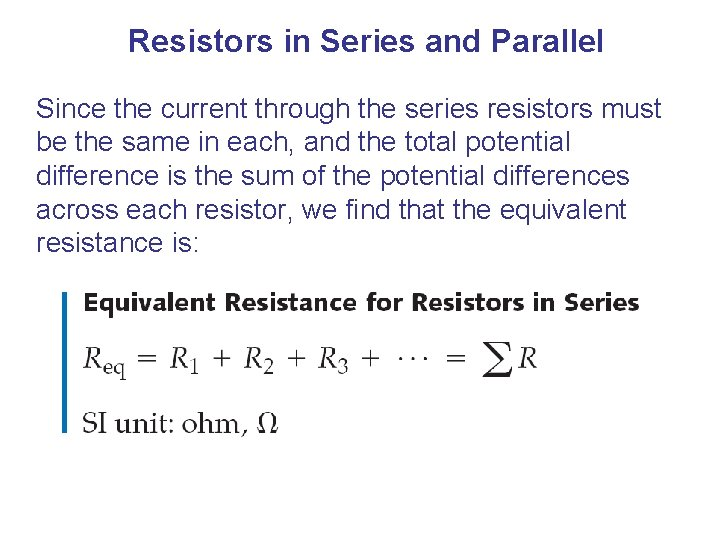Resistors in Series and Parallel Since the current through the series resistors must be the same in each, and the total potential difference is the sum of the potential differences across each resistor, we find that the equivalent resistance is:Resistors in Series and Parallel Resistors in parallel: are across the same potential difference; they can again be replaced by a single equivalent resistance: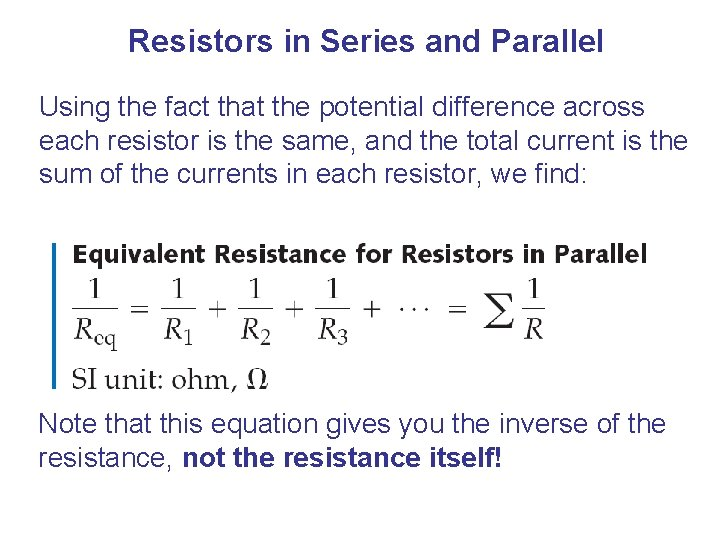Resistors in Series and Parallel Using the fact that the potential difference across each resistor is the same, and the total current is the sum of the currents in each resistor, we find: Note that this equation gives you the inverse of the resistance, not the resistance itself!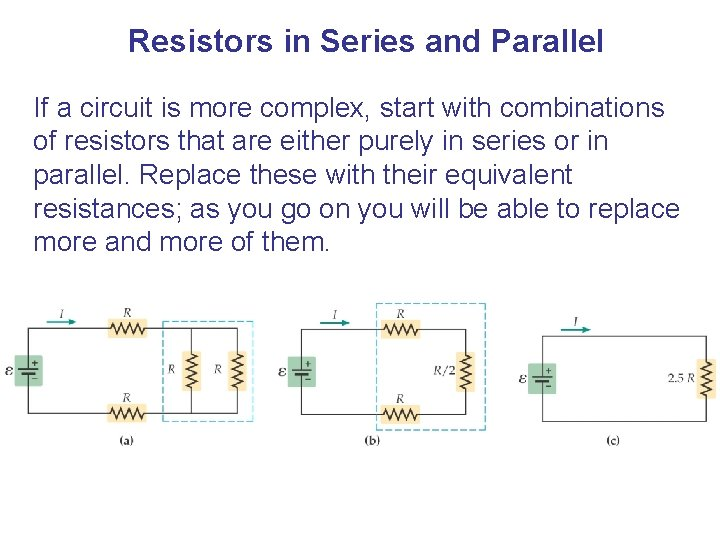Resistors in Series and Parallel If a circuit is more complex, start with combinations of resistors that are either purely in series or in parallel. Replace these with their equivalent resistances; as you go on you will be able to replace more and more of them.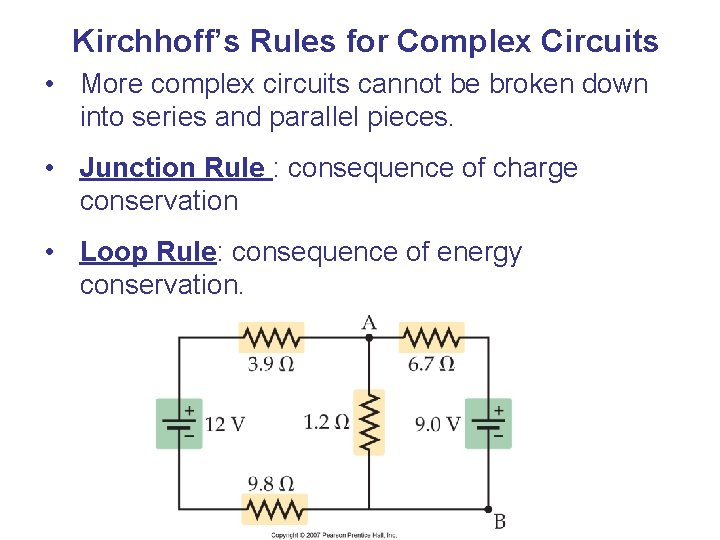Kirchhoff’s Rules for Complex Circuits • More complex circuits cannot be broken down into series and parallel pieces. • Junction Rule : consequence of charge conservation • Loop Rule: consequence of energy conservation.Kirchhoff’s Rules Junction Rule: At any junction, the current entering the junction must equal the current leaving it.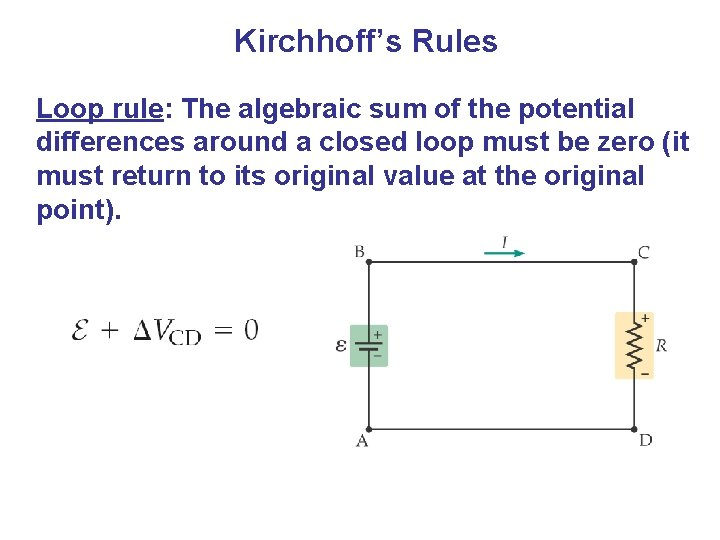Kirchhoff’s Rules Loop rule: The algebraic sum of the potential differences around a closed loop must be zero (it must return to its original value at the original point).Kirchhoff’s Rules Using Kirchhoff’s rules: • The variables for which you are solving are the currents through the resistors. • You need as many independent equations as you have variables to solve for. • You will need both loop and junction rules.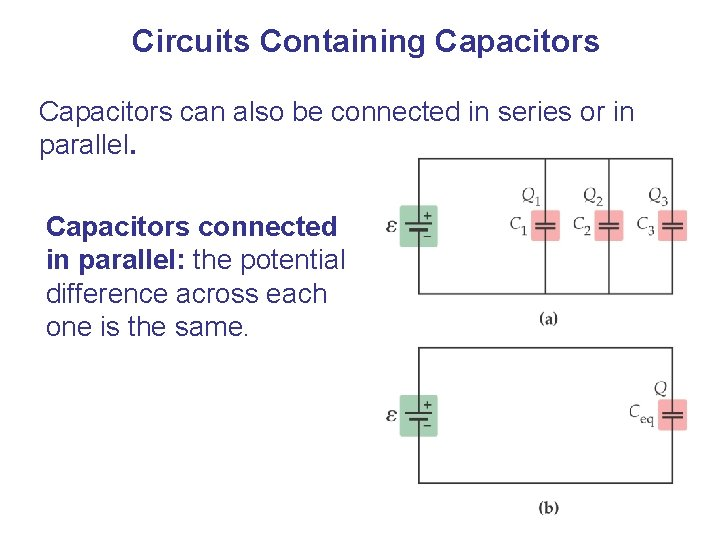Circuits Containing Capacitors can also be connected in series or in parallel. Capacitors connected in parallel: the potential difference across each one is the same.Circuits Containing Capacitors Therefore, the equivalent capacitance is the sum of the individual capacitances: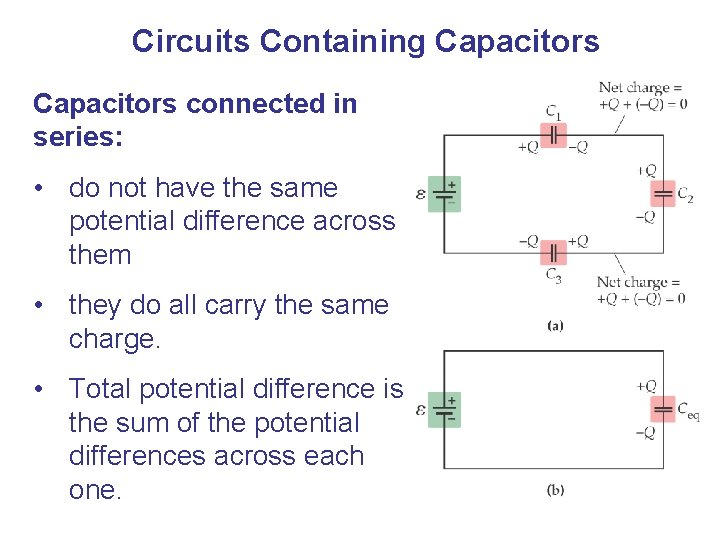Circuits Containing Capacitors connected in series: • do not have the same potential difference across them • they do all carry the same charge. • Total potential difference is the sum of the potential differences across each one.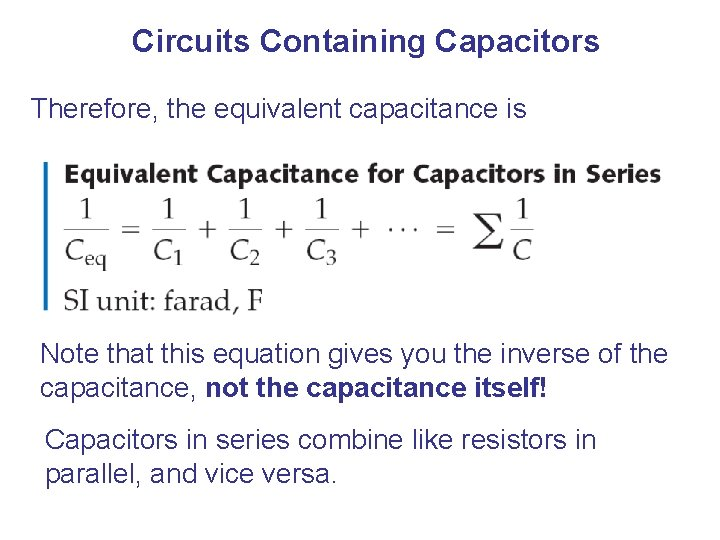Circuits Containing Capacitors Therefore, the equivalent capacitance is Note that this equation gives you the inverse of the capacitance, not the capacitance itself! Capacitors in series combine like resistors in parallel, and vice versa.RC Circuits In a circuit containing only batteries and capacitors, charge appears almost instantaneously on the capacitors when the circuit is connected. However, if the circuit contains resistors as well, this is not the case.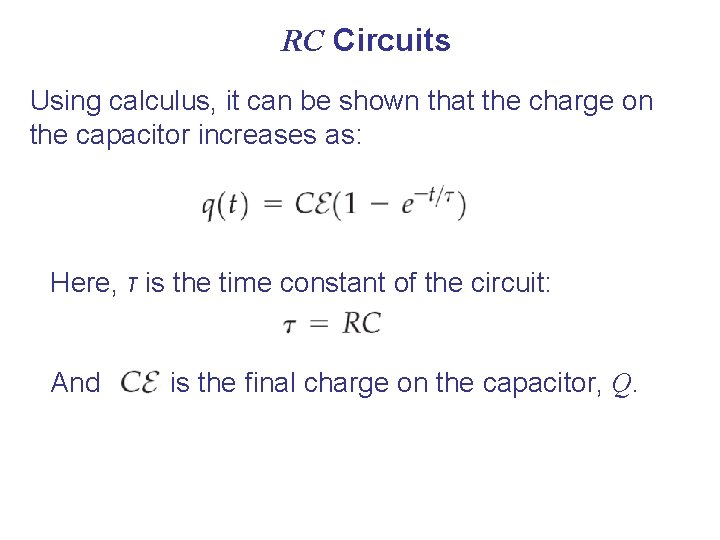RC Circuits Using calculus, it can be shown that the charge on the capacitor increases as: Here, τ is the time constant of the circuit: And is the final charge on the capacitor, Q.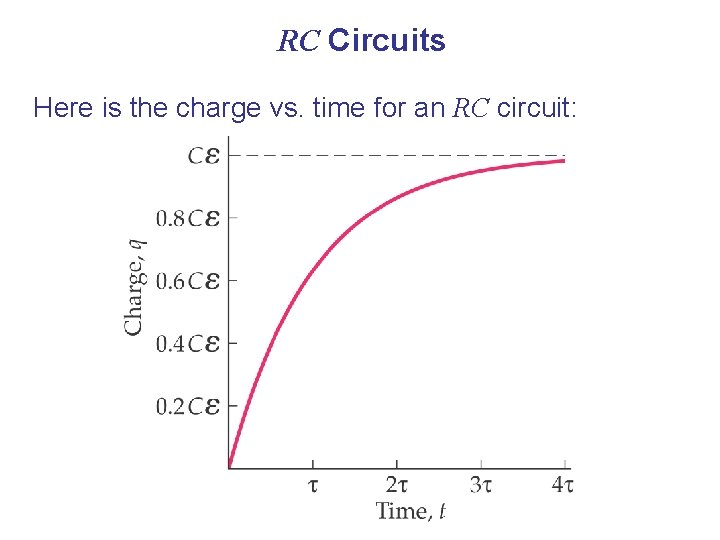RC Circuits Here is the charge vs. time for an RC circuit: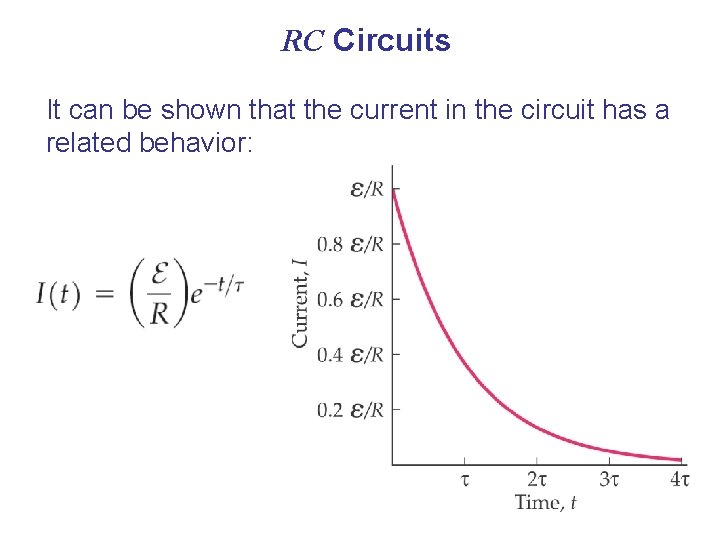RC Circuits It can be shown that the current in the circuit has a related behavior:Ammeters and Voltmeters An ammeter is a device for measuring current, and a voltmeter measures voltages. The current in the circuit must flow through the ammeter; therefore the ammeter should have as low a resistance as possible, for the least disturbance.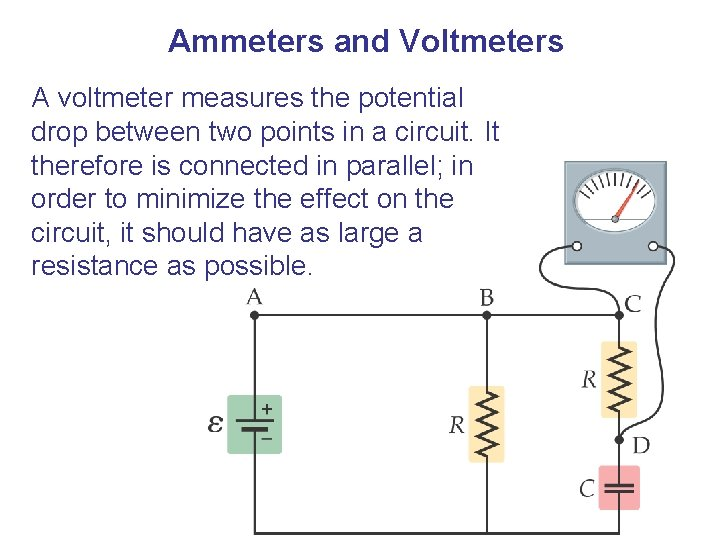Ammeters and Voltmeters A voltmeter measures the potential drop between two points in a circuit. It therefore is connected in parallel; in order to minimize the effect on the circuit, it should have as large a resistance as possible.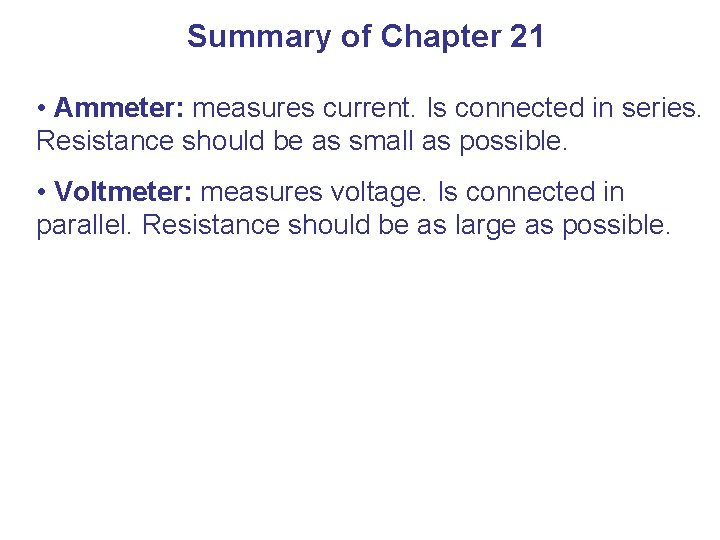Summary of Chapter 21 • Ammeter: measures current. Is connected in series. Resistance should be as small as possible. • Voltmeter: measures voltage. Is connected in parallel. Resistance should be as large as possible.